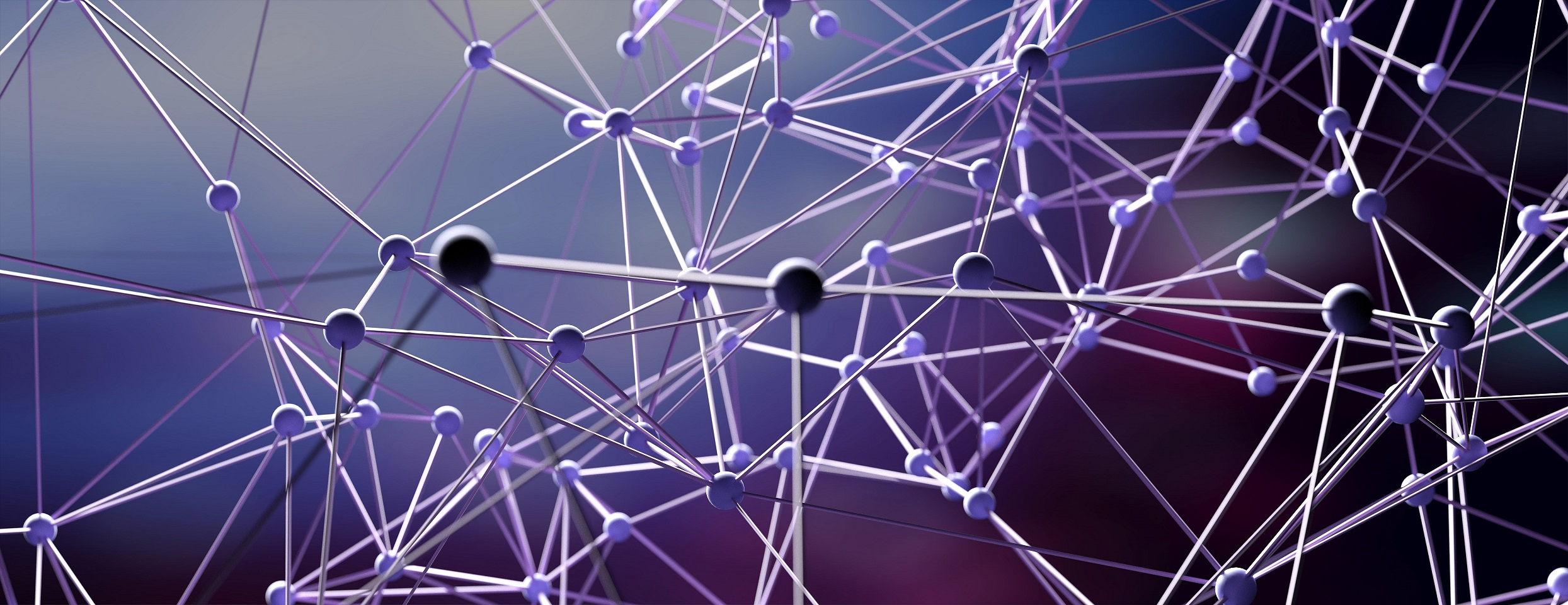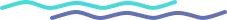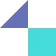# Backpropagation in Neural Networks

### What is Backpropagation in Neural Networks?

Backpropagation is a cornerstone of neural networks and is a powerful technique for training deep neural networks. It is a supervised learning algorithm used to train neural networks by making small adjustments to the weights of the connections between the neurons in the network.

With backpropagation, the network can learn from data and can make predictions. It works by propagating errors backward from the output layer of the network to the hidden layers and then adjusting the weights in the connections to reduce the overall error. This process is repeated until the network converges to a point where the prediction error is minimized. This technique has been used in many applications such as image recognition, speech recognition, natural language processing and robotics.

### How Does Backpropagation Work?

Backpropagation requires a known set of data representing the desired output.

It then processes the neural network one layer at a time, starting with the output layer and moving backward to the input layer.

At each layer, errors are calculated between the desired output and the actual output. The errors are then propagated back through the layers of the network and used to adjust the weights of the neurons.

This process is repeated until the desired output is achieved.

The backpropagation algorithm is an efficient approach to supervised learning.

It is also a powerful tool for training multilayer neural networks because it allows the network to learn complex nonlinear relationships between its inputs and outputs. By adjusting the weights of the neurons, the network can learn to recognize patterns in the input data and produce the desired output.

Backpropagation is a widely used algorithm in the field of artificial neural networks. It is an algorithm used to train these networks to recognize patterns and produce outputs that correspond to a given set of inputs.

Backpropagation has the advantage of being a simple and efficient algorithm which can be used to train networks with a large number of layers or nodes.

It is also well-suited for networks that require a lot of training data and have multiple layers. This makes it well-suited for many machines learning tasks.

On the downside, backpropagation can be computationally expensive and can take a long time to train a network.

It also tends to be very sensitive to the initial weights assigned to the nodes, meaning that the parameters need to be carefully tuned in order to get the optimal results.

Furthermore, backpropagation is prone to overfitting, meaning that the trained network will not generalize well to unseen data.

Finally, backpropagation is not well-suited for highly non-linear problems since it relies on gradient descent, which is not very effective in this case.

Despite these drawbacks, backpropagation is still a very powerful and useful algorithm. It has been used to achieve great success in many machine learning tasks and is still the most popular algorithm for training neural networks.

It is important to note that there are other algorithms that can be used for training neural networks, such as genetic algorithms and reinforcement learning, so it is always worth exploring these options if backpropagation is not providing the desired results.

### Understanding the Math Behind Backpropagation

The underlying mathematics of backpropagation is based on the chain rule of calculus, which is used to calculate the derivative of a function with respect to its inputs.

The chain rule states that the derivative of a composite function can be expressed as the product of the derivatives of its component functions.

Therefore, in order to calculate the derivative of the error in the output of a neural network, we need to calculate the derivative of the output neurons with respect to their inputs.

Once this is done, we can use the chain rule to calculate the derivative of the error with respect to the weights of the neurons in the previous layer.

This process is repeated until we reach the input layer, and the derivatives calculated at each layer can be used to adjust the weights of the neurons in order to minimize the errors of the network.

This is the basic concept behind backpropagation, and it can be extended to more complex networks with multiple layers and non-linear activation functions.

By understanding the mathematics behind backpropagation, it is possible to create powerful and accurate neural networks that can be used to solve a wide variety of problems.

### Implementing Backpropagation with Code

To implement backpropagation with code, we can use a gradient descent algorithm.

Gradient descent is an optimization algorithm used to find the minimum of a cost function.

In this case, we are using the cost function to measure the error in the network’s output.

To calculate the gradient of the cost function, we use the chain rule to find the partial derivatives of the cost function with respect to each weight in the network.

We then use these partial derivatives to update the weights.

#### Example

In a neural network with two layers and two neurons, we can define the weights and biases as follows:

w1 = 0.2
w2 = 0.4
b1 = 0.3
b2 = 0.1
The cost function is defined as the mean squared error (MSE) between the expected output and the actual output.
Cost = (expected - actual)2

The derivatives of the cost function with respect to each weight are calculated using the chain rule.

dCost_dw1 = dCost_dOutput * dOutput_dw1
dCost_dw2 = dCost_dOutput * dOutput_dw2

We then define the forward pass of the network, which calculates the output of the network given an input:

output = sigmoid(w1*input + b1) + sigmoid(w2*input + b2)

We then calculate the error between the actual output and the expected output:

error = expected - output

Finally, we use the derivatives of the cost function to update the weights using a gradient descent algorithm.

w1 = w1 - learning_rate * dCost_dw1
w2 = w2 - learning_rate * dCost_dw2

By implementing backpropagation with code, we can train artificial neural networks to learn various tasks.

The algorithm works by propagating the error from the output layer of the network to the hidden layers of the network, and then adjusting the weights accordingly.

By using a gradient descent algorithm to update the weights, we can find the optimal weights and biases that minimize the cost function and thus improve the accuracy of the network.

### Applications of Backpropagation

Backpropagation is a widely used and powerful technique in the field of artificial neural networks (ANNs).

Backpropagation is used in many different applications, such as computer vision, speech recognition, natural language processing, robotics, and even medical diagnosis.

#### Computer Vision

Backpropagation can be used to train an ANN to recognize objects in an image.

For example, an ANN can be trained to recognize a specific type of object such as a car, a person, or a cat. The ANN will be trained by feeding it input images of the desired object and teaching it to recognize the object. The ANN can then be used to detect the same object in new images.

#### Speech Recognition

In this application, an ANN is used to recognize spoken words or phrases. The ANN is trained by feeding it audio recordings of spoken words and teaching it to recognize the words. The ANN can then be used to recognize spoken words in new audio clips.

#### Natural Language Processing (NLP)

Here, an ANN is used to recognize the meaning of a sentence or a phrase. The ANN is trained by feeding it textual data and teaching it to recognize the meaning of the text. The ANN can then be used to understand the meaning of new sentences or phrases.

#### Medical Diagnosis

Here, an ANN is used to detect diseases or other medical conditions. The ANN is trained by feeding it medical data and teaching it to recognize the diseases or conditions. The ANN can then be used to diagnose new patients.

### Troubleshooting Backpropagation Algorithms

When troubleshooting backpropagation algorithms, it is important to first understand the basics of neural networks and how they are trained.

It is also important to have a good understanding of the cost function and how it is used to measure the performance of the neural network.

Once these concepts are understood, it is important to determine what type of backpropagation algorithm is being used and how it is being used to update the weights of the network.

In some cases, the backpropagation algorithm is not converging to the best solution, meaning it is not minimizing the cost function.

In this case, it is important to identify the cause of the problem and determine how to adjust the algorithm to achieve better results. This may require adjusting the learning rate, number of iterations, or other hyperparameters of the algorithm.

Additionally, it is important to understand the data being used for training, as this may be the source of the problem.

Finally, it is important to monitor the performance of the algorithm during training and make any necessary adjustments. This may include changing the learning rate or number of iterations, as well as implementing regularization techniques or adding more layers to the neural network.

By monitoring the performance of the algorithm, it is possible to identify the cause of any errors and make the necessary changes to improve the performance.

### Comparing Different Backpropagation Algorithms

There are a variety of different backpropagation algorithms, each with their own advantages and disadvantages.

#### Standard Backpropagation Algorithm

The most common backpropagation algorithm is the Standard backpropagation algorithm, which is a supervised learning algorithm. It uses the gradient descent method to adjust the weights of the network based on the error it receives from the output layer.

This algorithm is simple to implement and is well-suited for shallow networks. However, it has some limitations, such as being unable to learn long-term dependencies or having difficulty with noisy data.

#### Resilient Propagation (Rprop) Algorithm

Another popular backpropagation algorithm is the Resilient Propagation (Rprop) algorithm. This algorithm is a supervised learning algorithm that uses the sign of the gradient to adjust the weights of the network.

This algorithm is less sensitive to noisy data than standard backpropagation and can learn long-term dependencies.

However, it is more complicated to implement and is not as efficient as standard backpropagation.

#### Levenberg-Marquardt (L-M) Algorithm

The Levenberg-Marquardt (L-M) algorithm is an unsupervised learning algorithm that uses a combination of gradient descent and the Newton-Raphson method.

This algorithm is more efficient than standard backpropagation and can also learn long-term dependencies. However, it is difficult to implement and is often more computationally expensive than other algorithms.

The Adaptive Moment Estimation (Adam) algorithm is a combination of the Rprop and L-M algorithms and is used to improve the performance of deep neural networks.

This algorithm is more efficient than either of the other two algorithms and can learn long-term dependencies.

However, it is also more complicated to implement and requires more computational resources.

In conclusion, there are a variety of different backpropagation algorithms that can be used to train neural networks. Each algorithm has its own advantages and disadvantages, so it is important to choose the right algorithm for the task at hand.

Advanced backpropagation techniques are an important area of research in the field of artificial neural networks. These techniques can be used to improve the accuracy, efficiency, and generalization of neural networks.

#### Improved Generalization

One of the most popular advanced backpropagation techniques is called momentum.

In momentum, the weights and biases are adjusted with a momentum term, which is a kind of inertia that helps the network learn more quickly.

Another advanced backpropagation technique is called adaptive learning rate, which adjusts the learning rate of the network based on its performance.

Other advanced techniques include dropout and weight decay, which both help to regularize the network, and various forms of regularization, which help to reduce the risk of overfitting.

#### Improved Accuracy

For example, batch normalization can be used to reduce the training time of the network, while dropconnect can be used to improve the generalization of the network.

Other techniques such as data augmentation and transfer learning can be used to improve the accuracy of the network without increasing the complexity of the network.

#### Improve Efficiency

For example, dropout can be used to reduce the memory usage of the network and stochastic gradient descent can be used to reduce the computational complexity of the network.

Furthermore, sparsity-inducing techniques such as sparse coding and sparse autoencoders can be used to reduce the number of parameters in the network and improve the efficiency of the network.

Backpropagation has revolutionized the field of machine learning and has opened up many possibilities for artificial intelligence research. With its robust and effective algorithm, backpropagation continues to be a key component of neural networks and machine learning today.

Tooliqa specializes in AI, Computer Vision, Deep Learning and Product Design UX/UI to help businesses simplify and automate their processes with our strong team of experts across various domains.

Want to know more on how AI can result in business process improvement? Let our experts guide you.

Reach out to us at business@tooli.qa.

## FAQs

Quick queries for this insight

What is backpropagation?Backpropagation is an algorithm used to train neural networks. It is a supervised learning method that calculates the error for each neuron and adjusts the weights in the network based on the error to minimize it.

How does backpropagation work?Backpropagation works by calculating the error in the output layer and then propagating it backward through the network in order to adjust the weights. The weights in the network are adjusted based on the error in order to reduce it and reach the desired output.

What are the advantages of using backpropagation?The advantages of using backpropagation are that it is effective in training neural networks, it is computationally efficient, and it can be used to train multiple layers in a network. Additionally, it is relatively easy to implement and can be used to train large datasets.

### Connect with our experts today for a free consultation.

Oops! Something went wrong while submitting the form.

## Subscribe to Tooliqa

Oops! Something went wrong while submitting the form.## Built for Innovators

DICEWith advanced-tech offerings designed to handle challenges at scale, Tooliqa delivers solid infrastructure and solutioning which are built for to meet most difficult enterprise-level needs.​